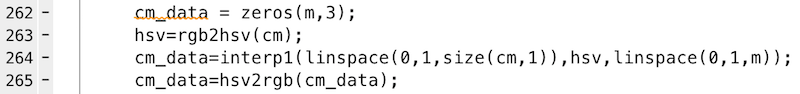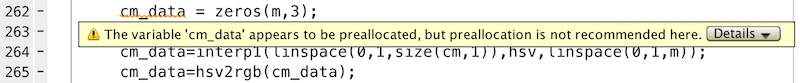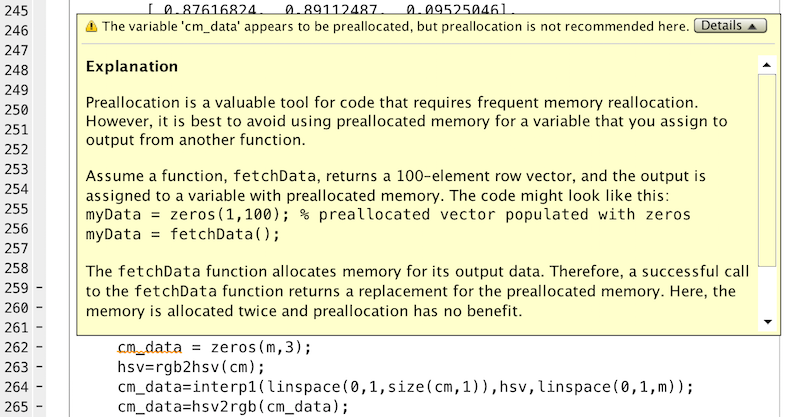# Ineffective preallocation

Today I want to explain a MATLAB coding mistake that I have seen even experienced MATLAB users make.

I was looking at some code on the File Exchange recently, and these lines caught my eye:

cm_data = zeros(m,3);
hsv=rgb2hsv(cm);
cm_data=interp1(linspace(0,1,size(cm,1)),hsv,linspace(0,1,m));
cm_data=hsv2rgb(cm_data);

Specifically, I was struck by the first and third lines. The first line creates an all-zeros matrix. Then the third line throws away the matrix created by the first line, replacing it with the output of interp1.

I have seen code like this before. It results from a misunderstanding of the concept of preallocation in MATLAB.

In MATLAB, preallocation refers to creating a matrix (usually with zeros) prior to entering a loop that would otherwise repeatedly resize the matrix.

Here is an example. In the for-loop below, the size of the matrix x is increased by one element each time through the body of the loop.

x = 0;
for k = 2:1000000
x(k) = x(k-1) + 5;
end


In versions of MATLAB before R2011a, this kind of loop could be quite slow. The reason is that MATLAB would reallocate the memory space for the matrix x each time through the loop, resulting in $O(N^2)$ execution time overall. To avoid this performance penalty, the usual recommendation was to create the full-size matrix x before entering the loop, like this:

x = zeros(1,1000000);
for k = 2:1000000
x(k) = x(k-1) + 5;
end


Although automatic array growth got much more efficient in MATLAB R2011a (it is no longer $O(N^2)$), there is still some performance benefit to preallocation, which is why it is still described in the documentation.

In the code from the File Exchange, though, the matrix cm_data is not being grown in a loop. The result of the initial assignment, cm_data = zeros(m,3), is just discarded without ever being used.

The MATLAB Editor tries to tell you about this. Do you see the orange squiggle on the first line below?If you hover over the squiggle with the mouse, you see this:And if you then click on the Details button, you get a full explanation:In summary, don't worry about putting in that call to zeros unless you are growing a matrix in a loop. If you later replace the variable with a completely new matrix returned by another function call, then the call to zeros is not helping you, and it might even slow down your code.

Published with MATLAB® R2016a

|

### コメント

コメントを残すには、ここ をクリックして MathWorks アカウントにサインインするか新しい MathWorks アカウントを作成します。